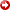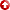--> --> Abstract: Parameter Estimation of Velocity Function in Unconsolidated Sand Via Semblance Velocity Analysis, by Abdullatif A. Al-Shuhail and Abdulrahman A. Al-Shuhail; #90077 (2008)
[First Hit]Parameter Estimation ofVelocityFunction in Unconsolidated Sand Via SemblanceVelocityAnalysis

Abdullatif A. Al-Shuhail* and Abdulrahman A. Al-Shuhail
KFUPM, Saudi Arabia
*[email protected]

Previous theoretical and field studies suggest the existence of continuousvelocity-depth functions in unconsolidated sand layers. One such function is V(z) = V0 √(1+kz1/3), where V0 and k are thevelocityfunction parameters which depend strongly on the layer porosity (F) and water saturation (Sw), and to a lesser extent, on the properties of the sand matrix, pore water and air. To properly understand seismic wave propagation in an unconsolidated sand layer, it is important to know itsvelocity-function parameters. This study proposes a procedure to estimate thevelocity-function parameters in unconsolidated sand layers.Velocity-function parameters are generally independent. However, it was possible to relate V0 and k of the above V(z) function because both parameters depended strongly on F and Sw. The relation   k(V0)=278 – 1.4x104/V0 + 4.9x105/V02     was found to fit k-V0 curves within 10% error for all F and Sw values lying within the ranges 0.1 < F < 0.65 and 0 < Sw < 0.9. Next, a table of V(z) functions corresponding to these F and Sw ranges was generated using F and Sw increments of 0.05. These V(z) functions were then used to calculate the corresponding time-offset (T-X) curves of the direct wave in the layer. The calculated T-X curves were fit to the observed T-X curve using the semblancevelocitymethod by scanning for the V0 value that generated the highest semblance value. The corresponding value of k was finally calculated using the above k(V0) relation. The procedure was successfully applied to a synthetic T-X dataset.

AAPG Search and Discovery Article #90077©2008 GEO 2008 Middle East Conference and Exhibition, Manama, Bahrain